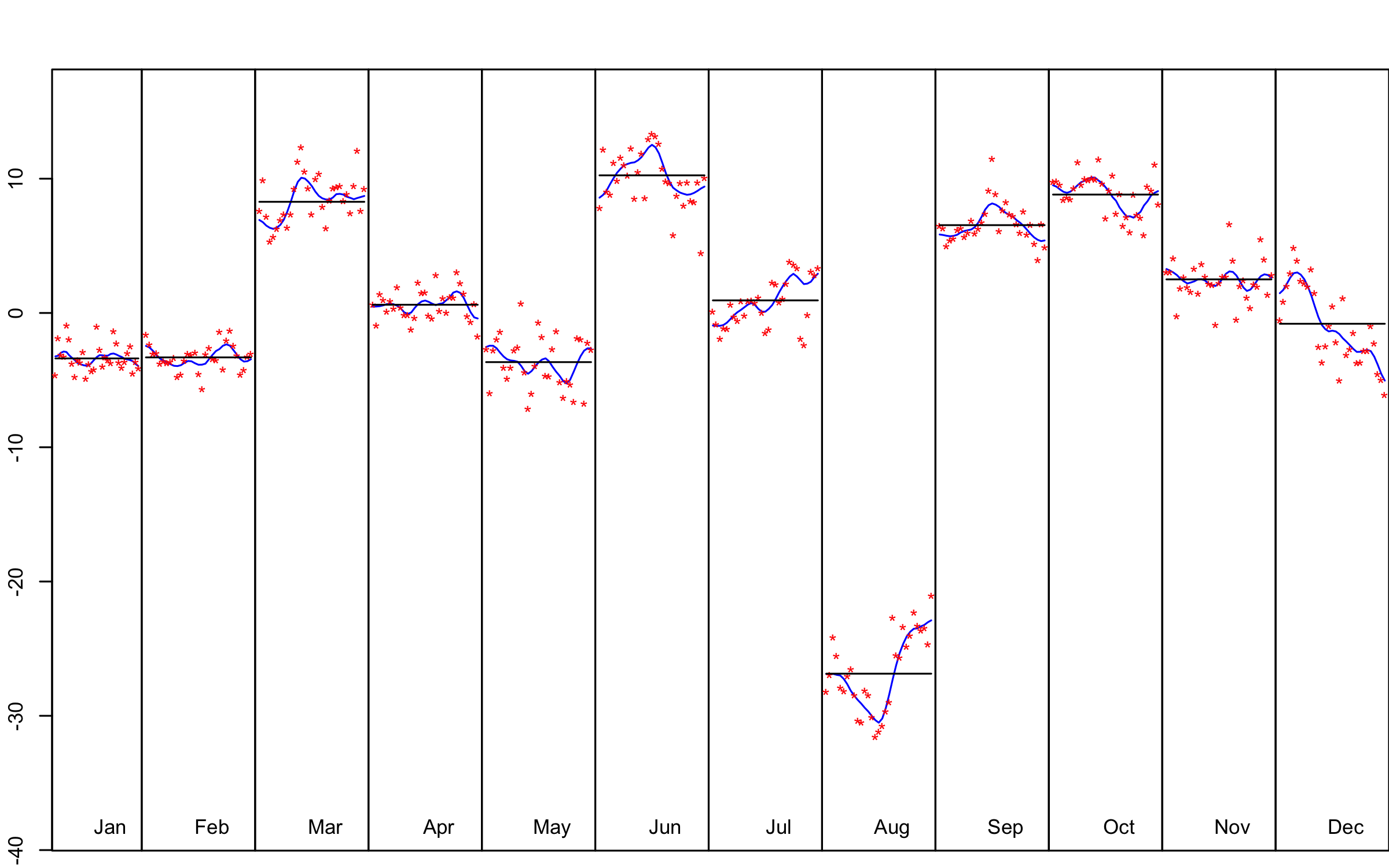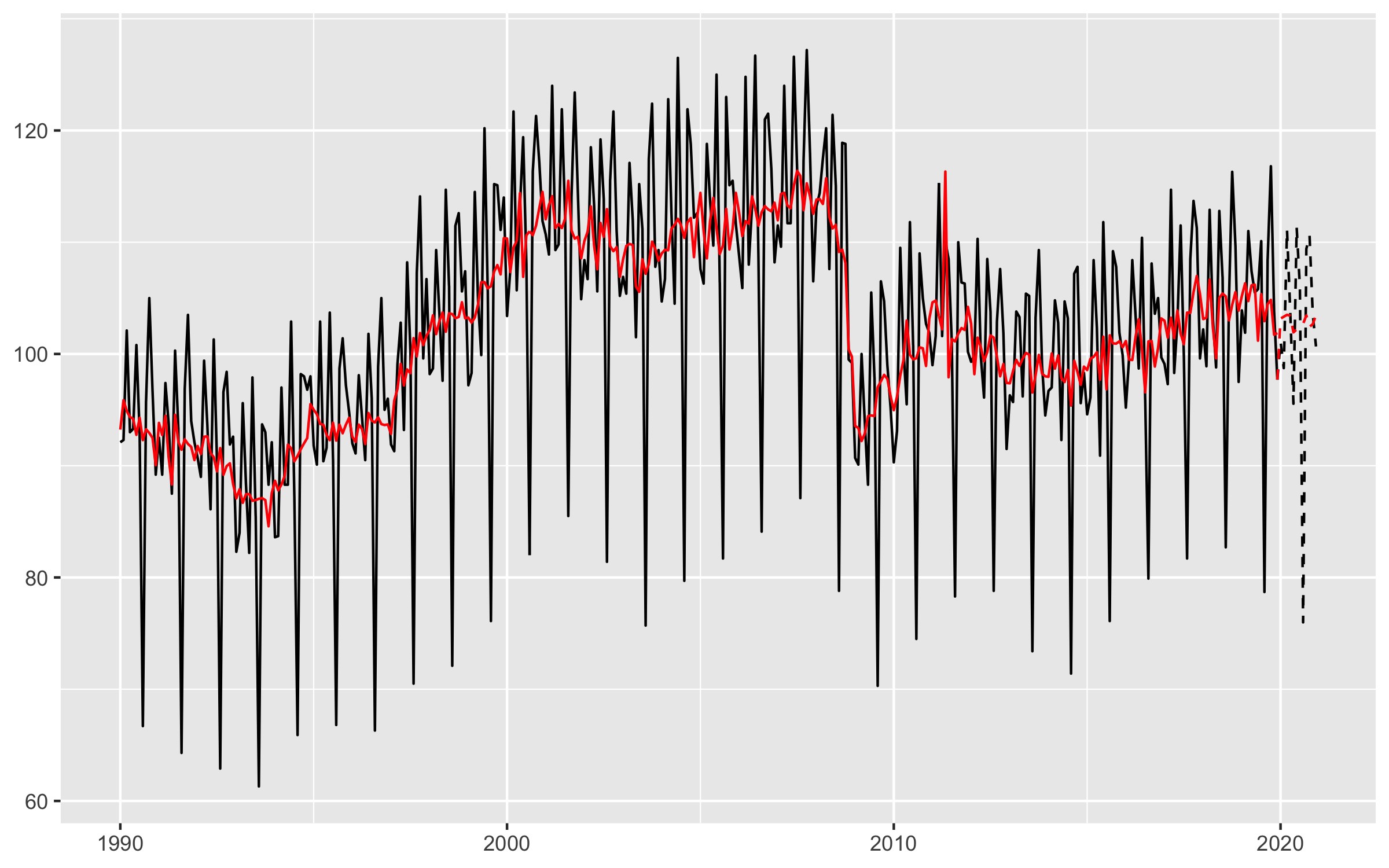# rjdmarkdown with HTML output

The functions developped in `rjdmarkdown` are:

• `print_preprocessing()` for the pre-processing model;
• `print_decomposition()` for the decomposition;
• `print_diagnostics()` to print diagnostics tests on the quality of the seasonal adjustment.

The result is different between X-13ARIMA and TRAMO-SEATS models.

``````library(rjdmarkdown)
library(RJDemetra)
sa_x13 <- x13(ipi_c_eu[, "FR"])
sa_ts <- tramoseats(ipi_c_eu[, "FR"])``````

# X-13-ARIMA model

``print_preprocessing(sa_x13)``

Pre-processing (RegArima)

Summary

372 observations

Easter  detected

4 detected outliers

Likelihood statistics

Number of effective observations = 359

Number of estimated parameters = 17

Loglikelihood = -799.084, AICc = 1633.964, BICc = 1.855

Standard error of the regression (ML estimate) = 2.218

ARIMA model

ARIMA coefficients
Coefficients Std. Error T-stat P (> | t|)
Phi(1) 0.000 0.108 0.003 0.998
Phi(2) 0.169 0.074 2.278 0.023
Theta(1) -0.549 0.102 -5.396 0.000 ***
BTheta(1) -0.666 0.042 -15.775 0.000 ***
Signif. codes: 0 ‘***’ 0.001 ‘**’ 0.01 ‘*’ 0.05 ‘.’ 0.1 ’ ’ 1
ARIMA (2,1,1)(0,1,1)

Regression model

Regression coefficientss
Coefficients Std. Error T-stat P (> | t|)
Monday 0.559 0.228 2.453 0.015
Tuesday 0.882 0.228 3.864 0.000 ***
Wednesday 1.040 0.229 4.535 0.000 ***
Thursday 0.049 0.229 0.215 0.830
Friday 0.911 0.230 3.964 0.000 ***
Saturday -1.578 0.228 -6.927 0.000 ***
Leap year 2.154 0.705 3.054 0.002 **
Easter  -2.380 0.454 -5.242 0.000 ***
TC (4-2020) -35.592 2.173 -16.377 0.000 ***
AO (3-2020) -20.890 2.180 -9.582 0.000 ***
AO (5-2011) 13.499 1.857 7.269 0.000 ***
LS (11-2008) -12.549 1.636 -7.673 0.000 ***
Signif. codes: 0 ‘***’ 0.001 ‘**’ 0.01 ‘*’ 0.05 ‘.’ 0.1 ’ ’ 1
``print_decomposition(sa_x13, caption = NULL)``

Decomposition (X-11)S-I Ratio

M-statistics
Value Description
M-1 0.163 The relative contribution of the irregular over three months span
M-2 0.089 The relative contribution of the irregular component to the stationary portion of the variance
M-3 1.181 The amount of period to period change in the irregular component as compared to the amount of period to period change in the trend
M-4 0.558 The amount of autocorrelation in the irregular as described by the average duration of run
M-5 1.020 The number of periods it takes the change in the trend to surpass the amount of change in the irregular
M-6 0.090 The amount of year to year change in the irregular as compared to the amount of year to year change in the seasonal
M-7 0.083 The amount of moving seasonality present relative to the amount of stable seasonality
M-8 0.244 The size of the fluctuations in the seasonal component throughout the whole series
M-9 0.062 The average linear movement in the seasonal component throughout the whole series
M-10 0.272 The size of the fluctuations in the seasonal component in the recent years
M-11 0.256 The average linear movement in the seasonal component in the recent years
Q 0.368
Q-M2 0.402
Final filters: M3x5, Henderson-13 terms
Relative contribution of the components to the stationary portion of the variance in the original series, after the removal of the long term trend
Component
Cycle 2.251
Seasonal 59.750
Irregular 1.067
TD & Hol. 2.610
Others 33.718
Total 99.395
``print_diagnostics(sa_x13)``
Diagnostics tests
P (> | t|)
mean 0.899
skewness 0.880
kurtosis 0.034
ljung box 0.000 ***
ljung box (residuals at seasonal lags) 0.212
ljung box (squared residuals) 0.024
qs test on sa 0.985
qs test on i 0.865
f-test on sa (seasonal dummies) 0.958
f-test on i (seasonal dummies) 0.893
Residual seasonality (entire series) 0.876
Residual seasonality (last 3 years) 0.906
f-test on sa (td) 0.987
f-test on i (td) 0.993
Signif. codes: 0 ‘***’ 0.001 ‘**’ 0.01 ‘*’ 0.05 ‘.’ 0.1 ’ ’ 1

# TRAMO-SEATS model

Some others graphics can also be added with the `ggdemetra` package, for example to add the seasonally adjusted series and its forecasts:

``````library(ggdemetra)
ggplot(data = ipi_c_eu_df, mapping = aes(x = date, y = FR)) +
geom_line() +
labs(title = NULL,
x = NULL, y = NULL) +
geom_sa(component = "y_f", linetype = 2,
frequency = 12, method = "tramoseats") +
geom_sa(component = "sa", color = "red") +
geom_sa(component = "sa_f", color = "red", linetype = 2)``````Seasonal adjustment of the French industrial production index

``print_preprocessing(sa_ts)``

Pre-processing (Tramo)

Summary

372 observations

Easter  detected

4 detected outliers

Likelihood statistics

Number of effective observations = 359

Number of estimated parameters = 11

Loglikelihood = -816.075, AICc = 1654.912, BICc = 1.852

Standard error of the regression (ML estimate) = 2.326

ARIMA model

ARIMA coefficients
Coefficients Std. Error T-stat P (> | t|)
Phi(1) 0.403 0.051 7.845 0.000 ***
Phi(2) 0.288 0.051 5.616 0.000 ***
BTheta(1) -0.664 0.042 -15.865 0.000 ***
Signif. codes: 0 ‘***’ 0.001 ‘**’ 0.01 ‘*’ 0.05 ‘.’ 0.1 ’ ’ 1
ARIMA (2,1,0)(0,1,1)

Regression model

Regression coefficientss
Coefficients Std. Error T-stat P (> | t|)
Week days 0.699 0.032 22.016 0.000 ***
Leap year 2.323 0.690 3.367 0.001 ***
Easter  -2.515 0.436 -5.773 0.000 ***
AO (5-2011) 13.468 1.787 7.535 0.000 ***
TC (4-2020) -22.213 2.205 -10.072 0.000 ***
TC (3-2020) -21.039 2.217 -9.492 0.000 ***
AO (5-2000) 6.739 1.794 3.757 0.000 ***
Signif. codes: 0 ‘***’ 0.001 ‘**’ 0.01 ‘*’ 0.05 ‘.’ 0.1 ’ ’ 1
``print_decomposition(sa_ts, caption = NULL)``

Decomposition (SEATS)